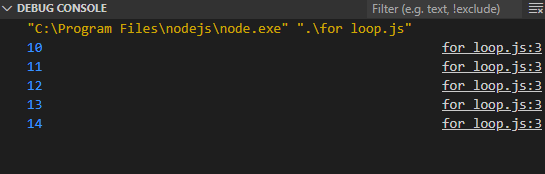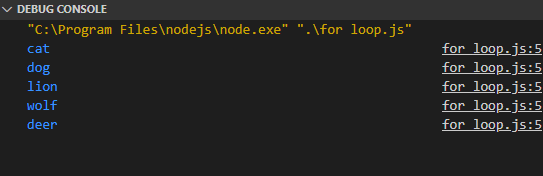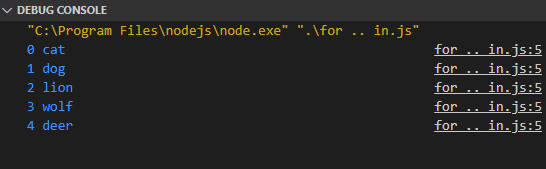GFG App
Open AppBrowser
Continue

# Explain the different variants of for loop in TypeScript

Loops are used to execute a specific block of code a specific number of times. There are 2 types of loops in TypeScript which are Definite Loop (for), and Indefinite Loops (while, do..while)

In TypeScript, we have basically 3 kinds of for loops.

• for
• for .. of
• for .. in

for loop: The for loop is used to execute a particular block of code for a specific number of times, which is defined by a specific conditional statement. This is a traditional for loop that we’ve been studying everywhere and using all the time.

Syntax:

```for(Initialization; Condition; Updation) {
...
}```
• Initialization statement executes before loop starts, and it initializes the iteration variable to a particular value, which is used to terminate the loop after certain iterations.
• Condition statement contains the termination condition which defines when the loop should stop. It is very important because an incorrect condition might cause the loop to continue forever!
• Updation statement is executed at the end of each iteration. It updates the value of the iteration variable. It is done so that the iteration variable reaches a value that falsifies the iteration condition thereby terminating the loop.

Example: Here, let i =10; is the Initialization statement that initializes an iteration variable i with an initial value of 10. The i < 15; is the condition that is checked before each iteration and i++; is the updation statement that increments the iteration variable i by +1 after each iteration.

## HTML

 `<``script``> ` `    ``for(let i = 10; i < ``15``; i++) { ` ` `  `        ``// This statement is repeated ` `        ``console.log(i); ` `    ``} ` ``

Output:Output of the code.

Example 2: In this example, we’ll create an array of some elements, and we will access each element of the array using the for loop.

## HTML

 `<``script``> ` `    ``let animals = ['cat', 'dog', 'lion', 'wolf', 'deer'] ` `     `  `    ``for(let i = 0; i < ``animals.length``; i++) { ` ` `  `        ``// Prints i-th element of the array ` `        ``console.log(animals[i]); ` `    ``} ` ` `

Output:Elements of the array are printed.

for…of loop: This is another type of for loop in Typescript that works in a different way. It operates on an iterable objects like arrays, lists, etc. It iterates each element of the iterable and assigns it to the iteration variable. So, there’s no need to write the traditional way of for loop if we want to access elements of an iterable. We can instead use for..of loop.

Syntax:

```for (initializer of collection) {
...
}```

Example: Here, the elements of the array animals are accessed one by one and are stored in the iteration variable i. So, i store the element of the array at a particular iteration, so we don’t need to access array elements manually. Also, the loop executes the same number of times as the length of the array, so we didn’t even have to worry about the termination condition.

## HTML

 `<``script``> ` `    ``let animals = ['cat', 'dog', 'lion', 'wolf', 'deer'] ` `     `  `    ``for(let i of animals) { ` ` `  `        ``// Print each element of the array ` `        ``console.log(i); ` `    ``} ` ``

Output:Elements of the array are printed.

The for..of loop is useful when we just want the elements of an iterable (array, list, tuple), and we don’t have to worry about the index of the elements inside the array.

for…in loop: The for..in loop works in a similar way as that of for..of loop, but instead of assigning the array elements to the iteration variable, the elements’ indices are assigned, so we get the element index at each iteration which can be further used to access individual array elements or perform necessary operations that require index instead of array elements (for example swapping of two elements).

Syntax:

```for (initializer in collection) {
...
}```

Example: Here, i is the iteration variable, that gets assigned to of the indices of the array one by one, starting from the first index that is 0 and going all the way up to the last index of the array which in this case is 4. The condition and updation are managed automatically by JavaScript.

## HTML

 `<``script``> ` `    ``let animals = ['cat', 'dog', 'lion', 'wolf', 'deer'] ` `     `  `    ``for(let i in animals) { ` `        ``// Print each element of the array ` `        ``console.log(i, animals[i]); ` `    ``} ` ``

Output: As in the code, I’ve printed i as well as animals[i], we get index and value at that index in each iteration.Index and value at index gets printed.

Conclusion: All the 3 for loops have their own advantages. Almost everything can be done using the traditional and universal for loop, but if we are working with iterables (arrays, lists, etc.), then using the specialized versions of for loop, i.e, for..of and for..in can result in cleaner and less complex code.

My Personal Notes arrow_drop_up